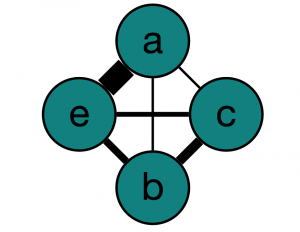# Yen’s k shortest paths

This week, we split up to delve further into the PathLinker paper according to our aims. I was assigned to research Yen’s k-shortest paths algorithm and present to the lab on Wednesday.

I’m now going to attempt to explain this algorithm–bear with me.

Some caveats: first, this is an algorithm for computing the k-shortest loopless paths from one node to another, and this algorithm only considers simple paths, where nodes may not be repeated.

To compute the k-shortest paths (where k is a user-defined parameter, say 10 or 4), we first find the shortest path (k=1) using some sort of algorithm, say Dijkstra’s.

Here’s our wonderful graph from Graphspace:Start Node End Node Weight
a b 1
a c 1
a e 9
b e 3
b c 4
c e 2

So, using our graph, let’s find the shortest path from a to e (P_1). Looking at the graph, we can see that it is a->c->e, with cost 4. Now what we do is loop through all paths using this first path as a guide, because intuitively, there might be some obvious edges that we use in many of the shortest paths.

Let’s call what we’re using from P_1 the root path, and call what we use when we diverge from it the spur path. To find P_2 (the 2nd shortest path), we must go through the graph differently this time to make sure we just don’t get the shortest path again. Because we’re using P_1 as a guide, let’s pretend that the edge from a->c has infinite cost, so that we can never take that edge.

The paths that result from the root path being (a) is then [a ->b->e (4), a->e(9), a->b->c->e(7)].

Then, we expand our root path to be a->c,  and pretend that the edge c->e has infinite cost, so the next path is [a->c->b->e (8)].

We combine the two lists of potential paths and look at their costs. [a->b->e (4), a->e(9),a->b->c->e(7), a->c->b->e(8)]. a->b->e is the 2nd shortest path (P_2)!

Then, we can simply search through the rest of the list for the next shortest path (P_3,…,P_k).